# The PML Visualization Projectby David Dumas and François Guéritaud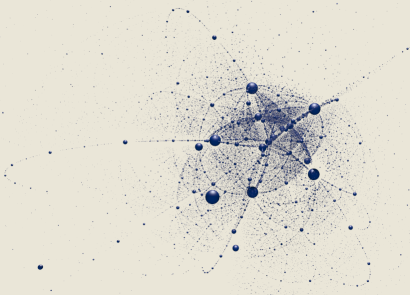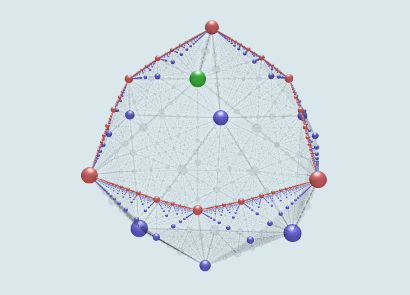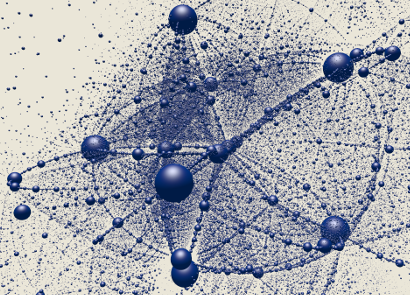### Introduction

In this project we study the space PML(S) of projective measured laminations on a surface S of negative Euler characteristic. Our goal is to make meaningful pictures of this space and its hierarchical structure using William Thurston's embedding of PML(S) into a cotangent space of Teichmüller space. In these visualizations, the points in PML(S) representing simple closed hyperbolic geodesics are marked by spheres; larger spheres correspond to shorter geodesics.

Thurston's embedding was introduced in the 1986 paper Minimal stretch maps between hyperbolic surfaces. Thurston showed that for any hyperbolic structure X on the surface, the map which takes a class [λ] in PML(S) to the covector dX(log Lλ) embeds PML(S) as the boundary of a convex neighborhood of the origin in the cotangent space TX*Teich(S). Here Lλ : Teich(S) → R is the hyperbolic length function.

For visualization purposes it is natural to consider cases in which the real dimension of the cotangent space of Teichmüller space is as small as possible. However, for closed orientable surfaces, this real dimension is at least six. We therefore consider non-orientable surfaces and surfaces with punctures.

### N3 — The sphere with three crosscaps

The non-orientable surface N3 of Euler characteristic -1 can be described as the connected sum of a sphere with three crosscaps (the "sphere model"), or as the connected sum of a torus and one crosscap (the "torus model"). The Teichmüller space of this surface has real dimension three, hence Thurston's embedding realizes PML(N3) as the boundary of a convex set in R3.

There are three types of simple closed curves on N3:

• The special one-sided curve fixed by the mapping class group (shown in green)
• Other one-sided curves (shown in blue)
• Two-sided curves (shown in red)
Examples of each class of simple curve can be seen in the figure below. Each curve is shown in both the torus model and the sphere model of N3.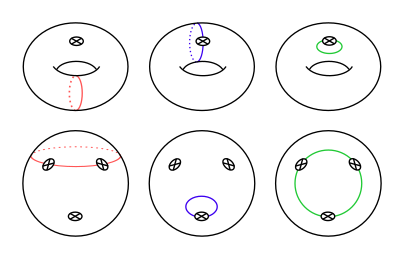In the torus description of N3, the special one-sided curve is the one that can be homotoped into the crosscap. The remaining simple curves on N3 come in dual pairs, with each pair consisting of a two-sided curve and a disjoint one-sided curve. Removing the crosscap, a dual pair becomes a simple closed curve and a disjoint simple arc on the one-holed torus; in this way, the set of dual pairs is in bijection with the set of simple curves on the torus.

A multi-curve on N3 is either the union of the two curves in a dual pair, a two-sided curve and the special one-sided curve, or the union of two or three disjoint one-sided curves. Examples are shown below.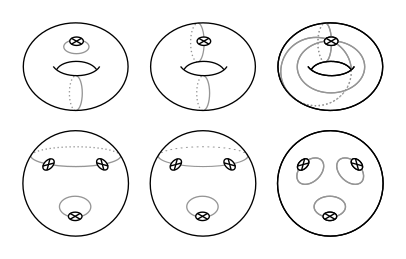To make a picture of Thurston's embedding, we mark the point in TX*Teich(N3)=R3 corresponding to a simple closed curve with a sphere whose radius is inversely proportional to the hyperbolic length of the corresponding geodesic. The spheres are colored according to the topological type of the associated curve as indicated above.

In the resulting pictures (see below), we see that the two-sided simple curves on N3 give a dense subset of a Jordan curve in two-dimensional sphere PML(N3). This equator curve is the projective measured lamination space PML(S1,1), which naturally embeds in PML(N3) through the torus picture described above. The one-sided curves in N3 dual to these two-sided simple curves accumulate on the equator from one side (i.e. they lie in one "hemisphere"). The other open hemisphere of PML(N3)) contains only one simple curve: the special one-sided curve.

#### Videos

• Rotating PML(N3)

• Earthquake path of PML(N3) embeddings

### N1,3 — The three-punctured projective plane

Next we consider finite area hyperbolic structures on the real projective plane (i.e. the crosscap) with three points removed. One way to picture this surface is to take a regular octahedron, remove the vertices, and take the quotient by the antipodal map. (This describes the six-punctured sphere as the orientable double cover of N1,3.) This construction also distinguishes a symmetric hyperbolic structure on N1,3, where the orientable double cover is conformally equivalent to the complement of the vertices of a regular octahedron in S2; we use this hyperbolic structure as the base point X for Thurston's embedding of PML(N1,3).

As before, the Teichmüller space has real dimension three, and there are one-sided simple curves, two-sided simple curves, and multi-curves. While PML(N1,3) is again homeomorphic a 2-sphere, it was shown by M. Scharlemann (see The complex of curves on non-orientable surfaces. J. London Math. Soc. 25 (1982) 171-184) that in this case the closure of the set of two-sided simple curves is an Apollonian gasket. In contrast, each one-sided curve is an isolated point in the set of simple curves; in fact, there is a single one-sided simple curve in each connected component of the complement of the gasket.

#### Videos

• Rotating PML(N1,3) in the octahedral Thurston embedding

### S0,5 — The five-punctured sphere

Finally we consider the case of finite area hyperbolic structures on the five-punctured sphere S0,5. In this case the Teichmüller space has real dimension four. In order to make pictures of Thurston's embedding, we first identify the cotangent space of Teich(S0,5) with R4 by choosing a basis. Then for each simple curve, we project the corresponding point in R4 radially onto the unit sphere S3, and then stereographically project into R3.

We use the "pentagonal" hyperbolic structure as the base point X for the Thurston embedding. This is the hyperbolic structure obtained by doubling a regular ideal pentagon across its boundary. We identify the tangent space TXTeich(S0,5) with R4 using a basis obtained from infinitesimal earthquake deformations along simple closed geodesics. In order to produce a symmetric picture, some care must be taken to choose a basis respecting the symmetry of the hyperbolic structure X. Specifically, we start with the blue and red geodesics shown below: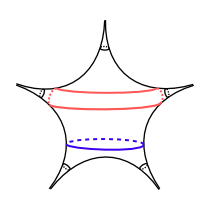Rotating the picture by multiples of 2π/5 we obtain two collections of five geodesics (blue and red). Infinitesimal earthquakes vectors along either collection span a 2-dimensional subspace of the tangent space, and the full tangent space splits as a direct sum of these two subspaces.

We therefore choose a basis {v1,v2,v3, v4} for the tangent space in which the blue earthquake vectors lie in the span of {v1,v2} and the red earthquake vectors lie in the span of {v3,v4}. Moreover the basis is chosen so that either collection forms the vertex set of a regular pentagon inscribed in the unit circle of its corresponding plane (considering the vectors vi to be orthonormal for the purposes of this geometric description).

After stereographic projection, we proceed as before, marking each of the resulting points with a sphere whose radius is inversely proportional to the hyperbolic length of the corresponding geodesic. Note that the sizes of the spheres are computed after stereographic projection: The length of the curve determines the Euclidean radius of the sphere drawn R3, rather than a spherical radius in S3. (While using spherical radii would seem more natural, the resulting pictures are cluttered and confusing. The few large spheres near infinity dominate the view and make it difficult to see the local structure near the origin.)

Despite the inherent distortion when using stereographic projection to visualize the boundary of this four-dimensional convex set, the intricate structure of PML and of the complex of curves is once again evident. Families of simple closed curves that lie in various four-punctured-sphere subsurfaces appear as a network of thin "filaments". Dehn twist families are seen approaching short curves from many directions.

#### Videos

Downloading the video gives the best quality, but the files are large. If watching on YouTube, some of the details will be blurry or "blocky". Select 720p HD viewing to minimize this.

#### Poster

This PDF poster shows two higher-quality renderings of 200,000 simple closed curves. In the top panel, all curves are represented by black spheres. In the bottom image, most curves are represented by 90%-transparent gray spheres, with only the curves which are disjoint from one of the five systoles highlighted in a bright color (with the color indicating which systole it is disjoint from). The systoles themselves are shown in black. The highlighted curves of any color form a circle, which is an embedded copy of the projective measured lamination space PML(S0,4).

The poster is intended for printing on "ARCH D" size paper (24in x 36in or 610mm x 914mm).

### Methods and tools

The basic process for generating these pictures is:
• Find explicit generators for the Fuchsian group corresponding to the base hyperbolic structure
• Compute a basis for the tangent space to Teichmüller space (as cocycles for a Fuchsian representation of the surface group into PSL(2,R))
• Generate a list of words in π1S corresponding to simple closed curves
• Compute lengths and length derivatives for these words
• Convert the length derivative data to a list of geometric primitives (centers and radii of spheres)
• Render animation frames and encode video files
Matrix generators for the Fuchsian group and representation cocycles representing the earthquake vectors were computed using elementary hyperbolic geometry. In some cases, Sage or Mathematica was used in these calculations.

Lists of words representing simple closed curves were generated by programs written in Python, by applying mapping class group elements to a set of "seed" words representing the different orbits. The visualizations shown above include 50,000 to 2,000,000 simple closed curves. The hyperbolic lengths and derivatives were then calculated by a finite difference method, resulting in data files representing Thurston's embedding.

A final set of Python programs handled stereographic projection, rotations, computing radii, and generating geometric primitives in the input format expected by the ray tracer POV-Ray. A separate template POV-Ray scene file set lighting, background, and texture parameters for the images and animations.

To create the videos, individual frame images were rendered with POV-Ray and then combined and encoded as h.264 video using FFmpeg. Rendering was the most time-consuming step in the process; each frame took between 5 and 30 minutes to render with a 3.7Ghz Intel Xeon E3-1290 V2 CPU.

### Source code

• The pmls05-demo repository on github has code samples from talks about the project and full code to generate five-punctured sphere images and animations.
• An older source release (Nov 2012) has code for generating the length derivative data for the five-punctured sphere, but lacks some documentation and corrections present in the github repository.
• (Planned: release source code for computing simple curves and length derivatives on the other surfaces, including N1,3 and N3.)
• ddencode is a simple front-end to FFmpeg to simplify the process of encoding a sequence of image files to a high-quality h.264 MP4 video file. It was used to generate the videos linked above (and the source videos for uploading to YouTube).

### Revision history

• 2017-05-21   –   PyCon video and slides
• 2017-05-17   –   CNRS and math institutes article links; corrections to description of methods
• 2017-05-16   –   Lecture video and github links
• 2016-06-23   –   Added PML(S0,5) poster
• 2015-09-30   –   Major update to include N3 and N1,3 visualizations, collaboration with F. Guéritaud
• 2012-11-29   –   PML(S0,5) Source code released.
• 2012-11-19   –   Re-encoded videos to address playback problems in Quicktime Player for Mac OS X; minor text edits.
• 2012-11-09   –   Added second rotation stereographic projection video.
• 2012-11-08   –   Initial release: Rotating stereographic projection video.

### Acknowledgements

Thanks to Saul Schleimer for helpful conversations related to this work.

This material is based upon work supported by the National Science Foundation. Any opinions, findings, and conclusions or recommendations expressed in this material are those of the author and do not necessarily reflect the views of the National Science Foundation.